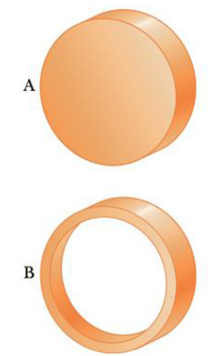Chapter 8.4, Problem 8.3QQ

Chapter
Section
Textbook Problem

The two rigid objects shown in Figure 8.21 have the same mass, radius, and angular speed, each spinning around an axis through the center of its circular shape. If the same braking torque is applied to each, which takes longer to stop? (a) A (b) B (c) more information is neededFigure 8.21 (Quick Quiz 8.3)

To determine
The time taken for the spinning objects to stop on application of same torque.

Explanation

The angular acceleration is given by,

α=τI

• I is the moment of inertia.
• τ is the applied torque.

Conclusion:

Moment of inertia of the hollow cylinder is greater than that of solid cylinder

Still sussing out bartleby?

Check out a sample textbook solution.

See a sample solution

The Solution to Your Study Problems

Bartleby provides explanations to thousands of textbook problems written by our experts, many with advanced degrees!

Get Started

Find more solutions based on key concepts